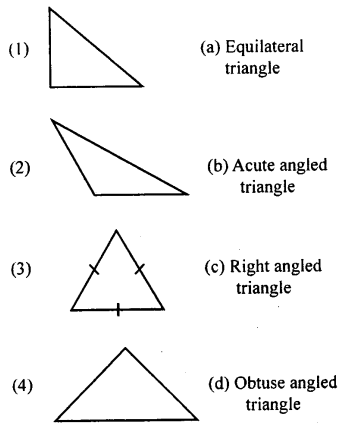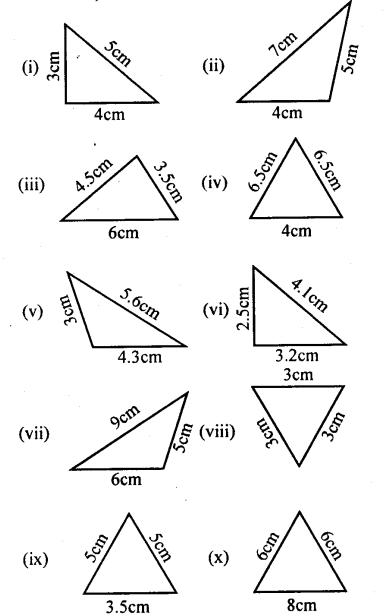# KSEEB Solutions for Class 8 Maths Chapter 6 Theorems on Triangles Ex 6.1

In this chapter, we provide KSEEB SSLC Class 8 Maths Chapter 6 Theorems on Triangles Ex 6.1 for English medium students, Which will very helpful for every student in their exams. Students can download the latest KSEEB SSLC Class 8 Maths Chapter 6 Theorems on Triangles Ex 6.1 pdf, free KSEEB SSLC Class 8 Maths Chapter 6 Theorems on Triangles Ex 6.1 pdf download. Now you will get step by step solution to each question.

## Karnataka Board Class 8 Maths Chapter 6 Theorems on Triangles Ex 6.1

Question 1.
Match the following1 – c
2 – d
3 – a
4 – b.

Question 2.
Based on the sides, classify the following triangles (figures not drawn of the scales)Equilateral triangle (viii)
Isosceles triangle (iv), (ix), (x)
Scalene triangle (i), (ii), (iii), (v), (vi), (vii).

All Chapter KSEEB Solutions For Class 8 maths

—————————————————————————–

All Subject KSEEB Solutions For Class 9

*************************************************

I think you got complete solutions for this chapter. If You have any queries regarding this chapter, please comment on the below section our subject teacher will answer you. We tried our best to give complete solutions so you got good marks in your exam.

If these solutions have helped you, you can also share kseebsolutionsfor.com to your friends.

Best of Luck!!